# Right Triangles

Right triangles are triangles in which one of the interior angles is 90 degrees, a right angle. Since the three interior angles of a triangle add up to 180 degrees, in a right triangle, since one angle is always 90 degrees, the other two must always add up to 90 degrees (they are complementary).

The side opposite the right angle is called the hypotenuse. The sides adjacent to the right angle are the legs. When using the Pythagorean Theorem, the hypotenuse or its length is often labeled with a lower case c. The legs (or their lengths) are usually labeled a and b.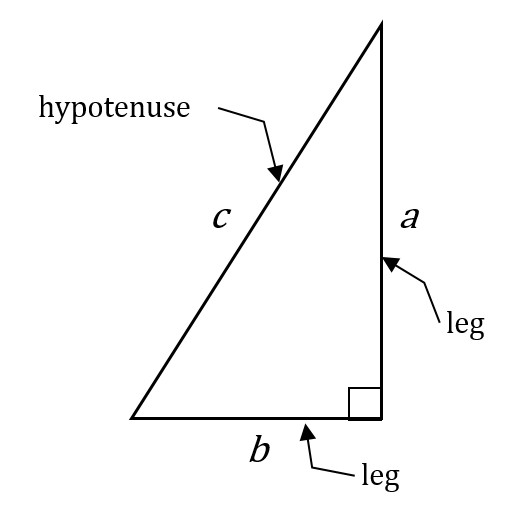Either of the legs can be considered a base and the other leg would be considered the height (or altitude), because the right angle automatically makes them perpendicular. If the lengths of both the legs are known, then by setting one of these sides as the base b and the other as the height h, the area of the right triangle is easy to calculate using the standard formula for a triangle’s area: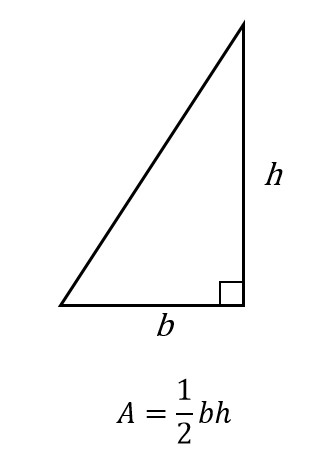This is intuitively logical because another congruent right triangle can be placed against it so that the hypotenuses are the same line segment, forming a rectangle with sides having length b and width h. The area of the rectangle is (b)(h), so either one of the congruent right triangles forming it has an area equal to half of that rectangle.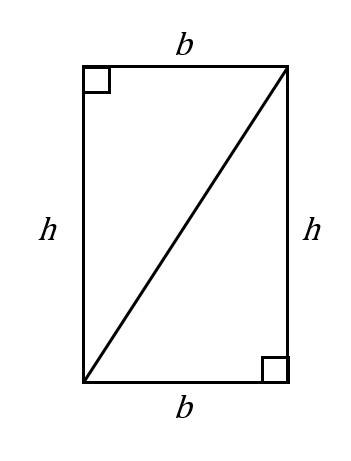## The Pythagorean Theorem

The Pythagorean Theorem states that, in a right triangle, the square of the length of the hypotenuse is equal to the sum of the squares of the lengths of the other two sides.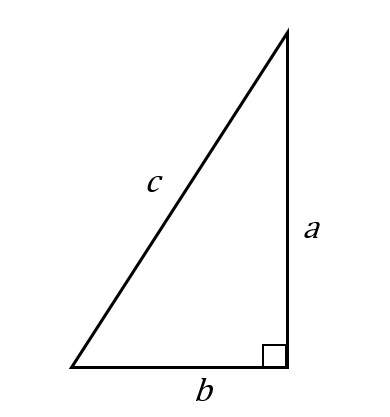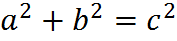The Pythagorean Theorem

The sides that we referred to as b and h above are now labeled as b and a, since the custom when using the Pythagorean Theorem is to employ the variables a, b, and c. Nevertheless, it’s important to recognize that both formulas refer to the same sides. For example, on a typical GMAT question you might use the Pythagorean Theorem to determine the base and height of a triangle and then ultimately find its area.

Quite frequently on the GMAT, you will find yourself in a situation in which you know that a particular triangle is a right triangle, and you will know the lengths of two of the three sides. You will then be able to find the length of the third side by plugging the two known lengths intoEarlier, we’ve discussed how the Pythagorean Theorem can be used to compute the distance between any two points in the coordinate plane.

## Common Pythagorean Triples

Certain trios of numbers that satisfy the Pythagorean Theorem appear often on the GMAT. You should memorize at least the first three triples here. You don’t need to know the angles of these triangles, only the ratios of the side lengths:On the GMAT, you are expected to know the angles and the side relationships for 45-45-90 and 30-60-90 triangles, but you are not expected to have memorized or to be able to determine the angles for Pythagorean triples listed above; you can determine the side lengths simply using the Pythagorean formula.

## 45-45-90 Triangle

Insofar as a right triangle is a special triangle, the 45-45-90 triangle is doubly special. All of the general triangle rules apply to it, the Pythagorean Theorem applies to it, and then it has some special properties of its own.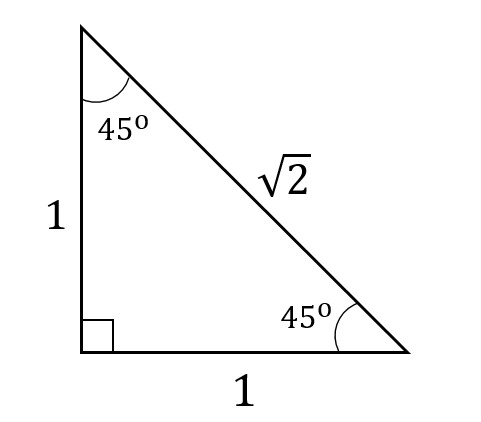A 45-45-90 triangle

A 45-45-90 triangle is what you get when you have an isosceles right triangle. If a triangle is a right triangle and it’s isosceles, then the two sides other than the right angle must add up to 90 degrees and they must be equal, so each must equal 45 degrees.

Furthermore, since it’s a right triangle, we know thatis true, but if a = b (because we are talking about an isosceles triangle), we can substitute that into the Pythagorean Theorem to get: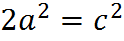If you try setting a = 1, then you get c = √2, as in the diagram above. The sides in every 45-45-90 triangle have lengths in the ratio 1: 1: √2.

The ratio of sides does not mean that the sides opposite the 45 degree angles are necessarily equal to 1, as they are above. Rather, whatever length is across from 45 degrees, call it a length of x, will be accompanied across from the hypotenuse by a side that is √2 as big, or x√2.

## 30-60-90 Triangle

The 30-60-90 triangle is similar to a 45-45-90 triangle, in that it is a “doubly special” right triangle. Also, as in the case of the 45-45-90 triangle, when we have one of these triangles, we know the measure of the angles and we know the ratio of the lengths of the sides.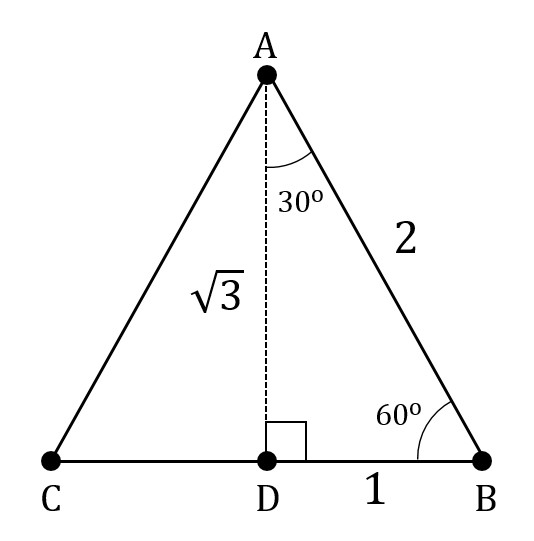A 30-60-90 triangle inside an equilateral triangle

A 30-60-90 triangle has angles that measure 30 degrees, 60 degrees, and 90 degrees, and sides facing these angles with lengths in the ratio 1:√3:2, respectively. As with any ratio, we can imagine that there is a missing number x, so that the lengths are x :x√3: 2x.

If we try plugging these lengths into the Pythagorean Theorem, we obtain the following: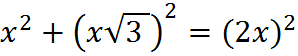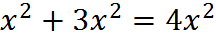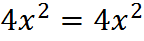Our result confirms that the theorem is true – as it had better be, since we are dealing with a right triangle.

You can remember which side measure goes across from which angle using the fact that greater side lengths in a triangle face greater angles. That means that the side across from the 30 degree angle will be the smallest side, the side across from the 60 degree angle will be the side of median length, and the side across from the 90 degree angle will be the longest side. When using this memory trick, sometimes people get tied up wondering whether √3 or 2 is greater. You can remember that √3 is smaller than 2 because 2 is equal to √4, and √3 is smaller than √4.

The figure above demonstrates a property that comes up sometimes on the GMAT: notice that the 30-60-90 triangle depicted is inside an equilateral triangle. By studying the diagram, you can convince yourself that any equilateral triangle contains two 30-60-90 triangles “hidden” inside it. That fact may appear within subtler geometry questions on the GMAT, so condition yourself to see two 30-60-90 triangles when an equilateral triangle is present.

## Trigonometry?

You do not need to know trigonometry for the GMAT. Remember sines, cosines and tangents of angles? Maybe you do and maybe you don’t, but you don’t need to know them for the GMAT. And, if you have studied these subjects and they are fresh in your mind, don’t be tempted to try to use them. Rather, look for another method to approach geometry questions using the material in this summary.